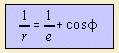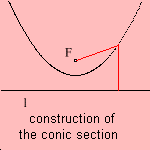# conic section

## mainA conic section can be defined as the intersection of a plane and a conic, that's where the name is from.
The relation between the angles of plane and conic determines the kind of conic section:

• equal: parabola
• smaller: ellipse; when the plane is parallel to the ground surface the resulting curve is a circle
• larger: hyperbola

These qualities were already known by Apollonius of Perga (200 BC). He wrote a series of books about the conic sections (Konika 1)), where he used work done by preceding scholars as Euclid (who was his teacher).
The first discovering of the conic sections has been made by Menaichmos (350 BC), a member of the school of Plato. He found the parabola on his attempts to duplicate the cube. But before that period, there was already a certain knowledge of the qualities of the conic sections, for instance in the head of Pythagoras (550 BC).

A conic section is an algebraic curve of the 2nd degree in x and y, and every 2nd degree equation represents a conic: the conic section is the quadratic curve.
The kind of conic section can be obtained from the coefficients of the equation in x and y.

The geometric treatment by the old Greek of the conic sections is equivalent with such an equation. But it had a different form, consisting of the theory of the 'adaptation of areas'. This theory can already be read in 'the Elements' of Euclid.
Depending the kind adaptation one of the three conic sections is obtained:Given a point F and a line l.
The conic section can be defined as the collection of points P for which the ratio 'distance to F / distance to l' is constant.
The point F is called the focus of the conic, the line l is called the directrix. The ratio is the eccentricity; its value gives the kind of conic section:

 eccentricity e conic section 0 circle 0 < e < 1 ellipse 1 parabola > 1 hyperbola

So the value gives the amount of deviation from a circle. When we set the distance of the focus to the line to 1, we can write for a conic section with eccentricity e the polar equation at top of this page. In this equation it is quite clear that the polar inverse is the limaçon.
The circular conic section is the circle.
The orbit of a comet can in fact take the form of each of the conic sections.

More detailed information about each of the conic sections:

A conic section is uniquely defined by 5 points, given the points are sufficient away from one line.
You can conclude this from the general equation of a conic through a point (x, y):
x2 + a y2 + b x y + c x + d y + e = 0.
This gives a linear equation in five variables (a to e), so five points define a conic.

notes

1) koonikos (Gr.) = cone